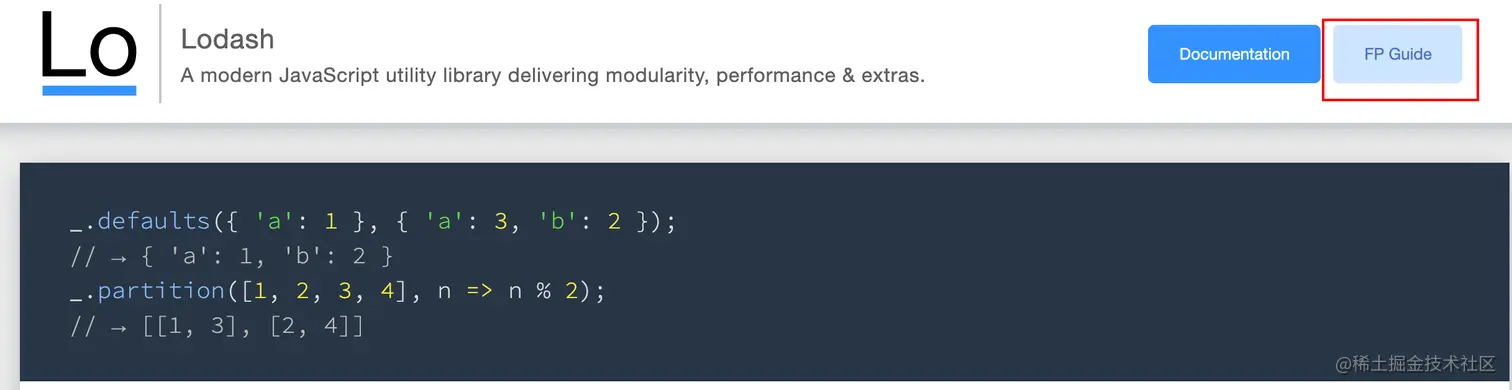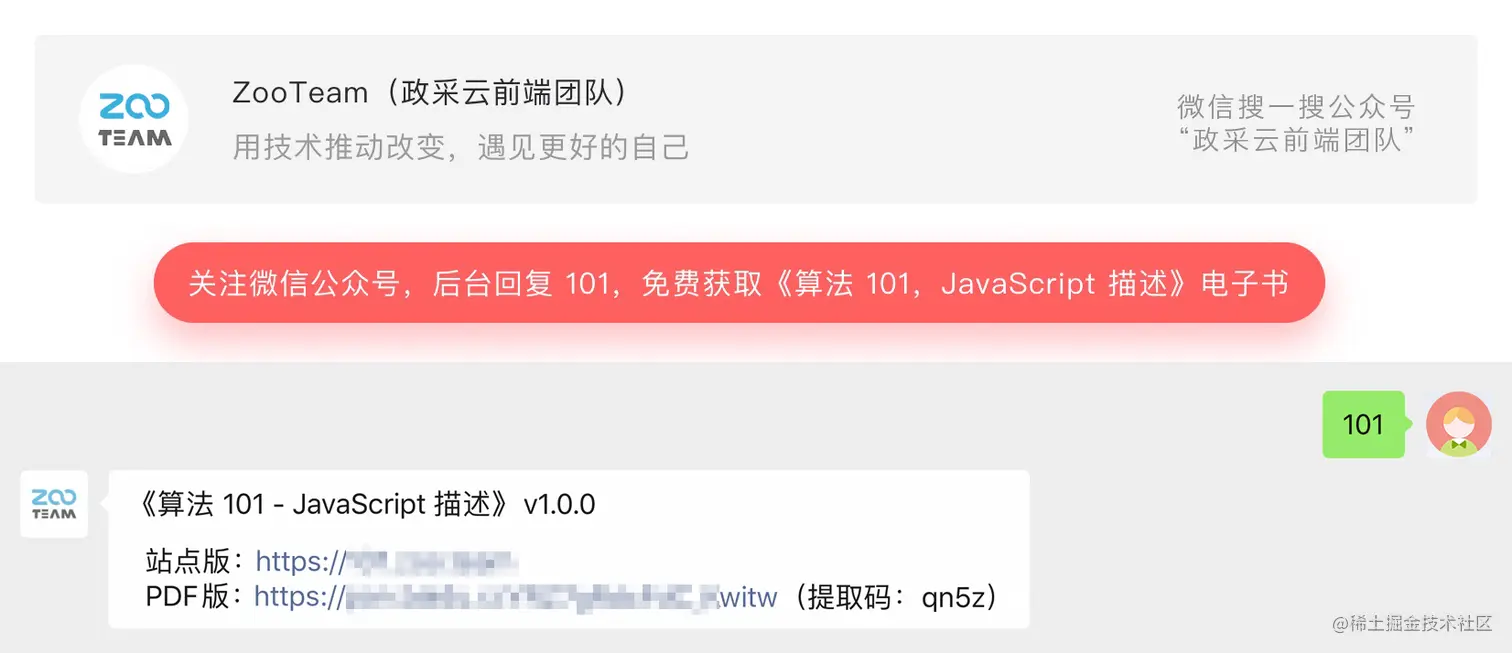# 函数式编程（FP）

5104## 写在前面

js 为了实现面向对象的思想，做了很多事情，导致大家在学习 js 的时候，会遇到复杂的原型、原型链、继承，还有对人不友好的 this ；而当我们用这些东西组合起来模拟面向对象的特性的时候，就更加痛苦了。但我们可以使用一种更友好的方式，函数式编程。

## 什么是函数式编程

• 面向过程编程：简单解释就是按照步骤来实现。

• 面向行为编程：它是函数式编程的衍生范型，将电脑运算平展为一系列的变化，并且避免使用程序指令以及堆叠的对象。

• 面向对象编程：它的思维方式是把现实世界中的事物抽象成程序世界中的类和对象，然后通过封装，继承和多态来演示事物之间的联系。

• 面向函数式编程：它的思维方式是把现实世界中的事物和事物之间的联系，抽象到程序世界中。

#### 函数式编程特点：

• 程序的本质：就是利用计算机的计算能力将输入转化成对应的输出

• 函数式编程中的函数指的不是编程语言里的函数，而是数学意义上的映射关系。比如 y=sin(x) 中 x 和 y 值的映射关系。

• 纯函数：相同的输入获得相同的输出（无副作用）。

• 函数式编程就是对**数据(函数)**映射关系的抽象。 举个例子：

y = \sqrt{a^{2}+b^{2}}

//非函数式 y = (a^2 + b^2)^0.5
const a = 3；
const b = 4;
const y = Math.sqrt(a*a + b*b)

//函数式 y = f(a, b)
const f = (a, b) => {
return Math.sqrt(a * a + b * b)
}
const y = f(3, 4)


#### 高阶函数 (high-order-function)

// 一个批量处理数组元素的例子
const use = ( arr, fn ) => {
return arr.map( i => fn(i))
}



#### 闭包 (closure)

function once(fn){
let done = false;
return function (){
if(!done){
done = true;
fn.apply(this, arguments)
}
}
}

const logOnce = once(console.log)

//此时只会执行一次
logOnce(1)
logOnce(1)


#### 纯函数 (pure function)

let numberArr = [1,2,3,4,5]
//纯函数
numberArr.slice(0,2) //[1,2]
numberArr.slice(0,2) //[1,2]
//不纯函数
numberArr.splice(0,2) //[1,2]
numberArr.splice(0,2) //[3,4]


• 对于耗时的操作，可对执行结果缓存，提高代码性能。
• 方便测试，降低排查问题的难度。
• 在多线程环境下（web worker），可对共享内存数据任意执行。

#### 柯里化 (currying)

const lucy = {age: 17}
const bob = {age: 100}

function checkAge(age){
return age > 18
}

checkAge(lucy.age)
checkAge(bob.age)


const lucy = {age: 17}
const bob = {age: 100}

function checkAge(min, age){
return age > min
}

checkAge(18, lucy.age)
checkAge(18, bob.age)


function checkAge(min){
return function(age){ // 函数作为返回
return age > min; // 闭包，引用外部参数
}
}

// 若果用es6的语法会更简洁
const checkAge = min => age => age > min

const checkAge18 = checkAge(18)

checkAge18(lucy.age)


#### lodash 中的 FP• 不可变
• 已柯里化（auto-curried）
• 迭代前置（iteratee-first）
• 数据后置（data-last） 假如我们有一个需求是将空格字符串以小写“ - ”分割该如何实现呢？

import _ from 'lodash'

const str = "CAN YOU FEEL MY WORLD"
const split = _.curry((sep, str)=>_.split(str, sep))
const join = _.curry((sep, arr)=>_.join(arr, sep))
const map = _.curry((fn, arr)=>_.map(arr, fn))

const f = _.flow(split(' '),map(_.toLower), join('-'))

f(str) //'can-you-feel-my-world'


import fp from 'lodash/fp'

const str = "CAN YOU FEEL MY WORLD"
const f = fp.flow(fp.split(' '),fp.map(fp.toLower), fp.join('-'))

f(str) //'can-you-feel-my-world'


• 不需要指明处理的数据
• 只需要合成运算过程
• 需要定义一些辅助的基本运算函数 当然使用的时候还是需要注意一下参数的描述。官网上有一个 🌰 是这样的：
// The lodash/map iteratee receives three arguments:
// (value, index|key, collection)
_.map(['6', '8', '10'], parseInt);
// ➜ [6, NaN, 2]

// The lodash/fp/map iteratee is capped at one argument:
// (value)
fp.map(parseInt)(['6', '8', '10']);
// ➜ [6, 8, 10]



FP 中的 map 方法和 lodash 中的 map 方法参数的个数是不同的。

#### 什么是函数组合withRouter(Form.create()(connect(({ model }) => ({ status: model.status}))(Index)))


withRouter
@Form.create()
@connect(({ model }) => ({
status: model.status,
}))
Index


function compose(...args){
return function(value){
return args.reverse().reduce(function(acc,fn){
return fn(acc)
}, value)
}
}
// es6
const compose = (...args) => value => args.reverse().reduce((acc,fn) => fn(acc), value)


const log = curry((label, x) => { console.log(label, x); return x; });
const y = compose(c, log('after a'),b, log('after a'), a);


#### 函子（Functor）

class Container{
constructor(value){
//私有的值 不对外公布
this._value = value
}
//接收一个处理值的函数
map(fn){// map 是一个契约名称 fn 需要是一个纯函数
//返回一个新的函子
return new Container(fn(this._value))
}
}

new Container(1)
.map(x => x + 1)
.map(x => x * x)


class Container{
//of 的作用就是给我们返回一个函子对象，我们把 new 关键字封装在里面
static of(value){
return new Container(value)
}

constructor(value){
this._value = value
}

map(fn){
return new Container(fn(this._value))
}
}
new Container.of(1)
.map(x => x + 1)
.map(x => x * x)


• maybe 函子: 空值问题
• Either 函子：异常处理
• IO 函子：副作用处理

## 主流框架、库中的应用

const middleware = store => next => action => {
// 具体实现
};


(store, next, action) => {...}


• 通过自定义 hooks 来共享一些组件的逻辑，如果用类组件实现，只能通过高阶组件模拟，这样会不断嵌套，无用的“龟壳”。
• 每个方法都是独立的, 不需要像类组件那样在一个 mount 生命周期里做一堆不相关的操作，更新时又做一堆不相关的操作。不相关的逻辑整合在一个生命周期内，本来就是不易读、不易维护的。
class Example extends Component {
componentDidMount() {
//注册事件
//请求Api
//设置状态 等等
}
componentWillUnmount(){
//取消一些监听事件
}
}


• 政采云前端小报

• 商品选择 sku 插件

## 招贤纳士••••••••